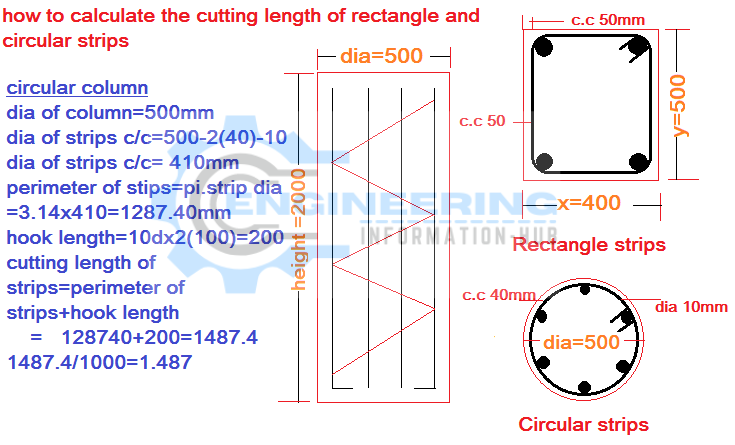Bar Bending Schedule

# How to Calculate the Cutting Length of Rectangle and Circular Stirrups

### How to Calculate the Cutting Length of the rectangle and Circular Stirrups

today I have the topic of how to calculate the cutting length of circular and rectangle stirrups. rectangle stirrups are used in the rectangle column and circular stirrups are used in the circular column.

Cut length mean total length which needs to be cut in steel.

we have given data of both column rectangle and circular as mentioned in below drawing### 2 How To Calculate The Bar Bending Schedule in Circular Slab

given data

length of the column  y =500mm

the breadth of the column  x=400mm

dia of stirrups bar =10mm

concrete cover=50mm

### solutioncutting length of stirrup y=500-2 concrete cover

cutting length of stirrup y =500-2(50)=400mm

cutting length of stirrup x= 400-2 concrete cover

cutting length of stirrup x=400-2(50)=300mm

hook length = 10d =10 x 10=100mm

cutting length of stirrups = x side ×2+ y side × 2+2 hooks length

cutting length of stirrups =(300×2)+(400×2)+2(100)

cutting length of stirrups =600+800+200=1600=1.6m

### cutting length of circular stirrups

given data

diameter of column=500mm

concrete cover of circular column=40mm

diameter of stirrups bar =10mm

diameter of  stirrup center to center= dia of column-2 concrete cover-dia of the stirrup bar

diameter of stirrup center to center=500-2(40)-10=410mm

hooks length of stirrups = 10d=10×10=100mm

the perimeter of stirrups =π×d

cutting length of stirrups = perimeter of stirrup+2hooks

cutting length of stirrups =3.14×410+2(100)

### Raja Numan

Hi, My name is Engr. Raja Numan author of Engineering Information Hub and I am a Civil Engineer by Profession and I've specialized in the field of Quantity Surveying, Land Surveying as QC Engineer in national and multinational companies of Pakistan & Saudi Arabia.

### Related Articles

1.fafaslot says: